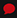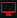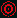Careers800-626-6653

# Springs FAQ

### Are there any calculations or recommendations available to assist me with selecting a Mold Spring?

Some customers call in for assistance in selecting mold and die springs offered by DME. We present the following method. If you require additional assistance, please do not hesitate to contact us.

Please note: the terms compression vs. deflection, and force vs. load are used interchangeably in this text.

The general formula for compression springs is

F = L * K where:

F = force or load [lb]L = deflection or compression [in]K = spring constant [lb/in]

Our catalog always lists the force at 1/10” compression, and we will call it DC (DME Constant). This force is actually 10% of standard K spring constant defined in engineering calculations. The Engineering K is the theoretical load at 1” deflection and the DC is the load at 0.1” deflection, so
K = 10 * DC

The DME catalog also lists the force at maximum allowable compression. The maximum compression is given as the percentage of the Free Length, although the length and percentage are seemingly inconsistent units.

The force at 0.1” compression is also marked with DC in the attached chart. The 50% Maximum Recommended Deflection means that the spring can be compressed by maximum 50% of the Free Length (we marked as FL in the chart), 30% deflection is when the spring is compressed by 30% of its Free Length. Since the force is proportional to the compression, the Force-Length graph is a straight line.

To better explain the numbers provided in the DME catalog, for example, let’s consider the SMD2030 spring as shown on page 343 in the DME Mold Components catalog:

• -spring-graph.jpg
• Catalog number: SMD2030

From the Catalog:

FL (Free Length) = 7.50 [in]DC = 1.2 [lb] (load at 0.1” deflection) => K = 10 * DC = 12 [lb/in]F50 = 45.0 [lb] (load at 50% deflection)

-catalog springs.jpg

Let’s double-check the F50 force given in the catalog by calculation:

L50 = 0.5 * FL = 0.5 * 7.5 = 3.75 [in] (50% deflection)This is 3.75 / 0.1 = 37.5 times more than the 0.1 [in] deflection, so the F50 force is 37.5 times larger than the force at 0.1” deflection:

F50 = (0.5 * FL) * K = 3.75 * 12 = 45.0 [lb]

If we want to know the force at a certain (different) deflection, we need to multiply this number with the K spring constant.

For example: What would the load (force) be at 2.65 [in] deflection?

F = 2.65 * K = 2.65 * 12 = 31.8 [lb]

Usually we need a certain Pre-Load in the fully extended position in order to have a minimum force. The basic force calculation is the same as above.
If we know the Travel (working compression) plus the Pre-Load, their sum is the Total Compression. The Total Compression should always be less than or equal to the maximum recommended deflection. The Total Compression (which is the Travel plus Pre-Load) divided by the percentage in the catalog gives you the minimum necessary Free Length. If this Free Length is available in the catalog, this is the spring you need to pick. If this calculated minimum Free Length is not available, you need pick the next longer standard Free Length.

Please note that if the calculated forces are acceptable, always try to use longer Free Length springs, so the Total Compression is less than the maximum allowable compression. This increases the useful life of the spring.

Another example (the same spring):